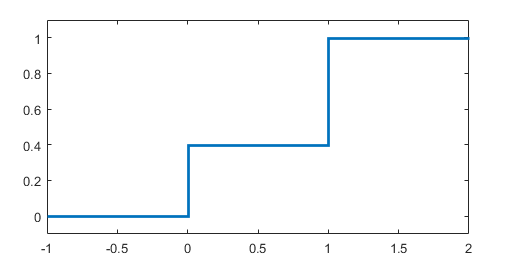15
Mar 17

## Examples of distribution functions

Examples of distribution functions: the first two are used in binary choice models, the third one is applied in maximum likelihood.

### Example 1. Distribution function of a normal variable

The standard normal distribution$z$ is defined by its probability density$p_z(x)=\frac{1}{\sqrt{2\pi}}\exp(-\frac{x^2}{2}).$

It is nonnegative and integrates to 1 (the proof of this fact is not elementary). Going from density function to distribution function gives us the distribution function (cdf) of the standard normal:$F_z(x)=\int_{-\infty}^xp_z(t)dt,$ for all real$x$.

### Example 2. The logistic distribution

Consider the function$F(x)=\frac{1}{1+e^{-x}}.$

It's easy to check that it has the three characteristic properties of a distribution function: the limits at the right and left infinities and monotonicity.

1. When$x\rightarrow\infty$$1+e^{-x}$ goes to 1, so$F(x)$ tends to 1.

2. If$x\rightarrow -\infty$$1+e^{-x}$ goes to$+\infty$, and$F(x)$ tends to 0.

3. Finally, to check monotonicity, we can use the following sufficient condition: a function is increasing where its derivative is positive. (From the Newton-Leibniz formula$f(x_2)=f(x_1)+\int_{x_1}^{x_2}f'(t)dt$ we see that positivity of the derivative and${x_2}>x_1$ imply$f(x_2)>f(x_1)$). The derivative

(1)$F'(x)=\frac{e^{-x}}{(1+e^{-x})^2}$

is positive, so$F(x)$ is increasing.

Thus,$F$ is a distribution function, and it generates a density (1).

### Example 3. Distribution function and density of a discrete variable

The distribution function concept applies to all random variables, both discrete and continuous. For discrete variables, the distribution function is not continuous as in Figure 1 here; it has jumps at points that have a positive probability attached. We illustrate this using a Bernoulli variable$B$ such that$P(B=0)=0.4$ and$P(B=1)=0.6$.

1. For$x<0$ we have$F_B(x)=P(B\le x)=0$.
2. For$0\le x<1$ we have$F_B(x)=P(B=0)+P(0.
3. Finally,$F_B(x)=P(B=0)+P(B=1)+P(1 for$1\le x<\infty$.

This leads us to Figure 1.Figure 1. Distribution function of the Bernoulli variable

Now consider$B$ such that$P(B=1)=p$ and$P(B=0)=1-p$. The analog of the density function for Bernoulli looks like this:

(2)$p(x)=p^x(1-p)^{1-x},$ for$x=0,1$.

To understand this equation, check that$p(1)=p$ and$p(0)=1-p$. In Math, there are many tricks like this.

Remark. For continuous random variables, the value of the density at a fixed point means nothing (in particular, it can be larger than 1). It is its integral that has probabilistic meaning. For (2) the value of the density at a fixed point IS probability.